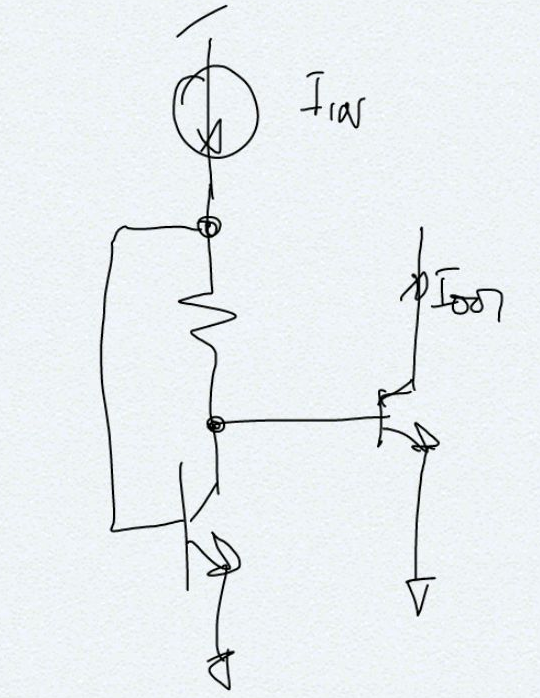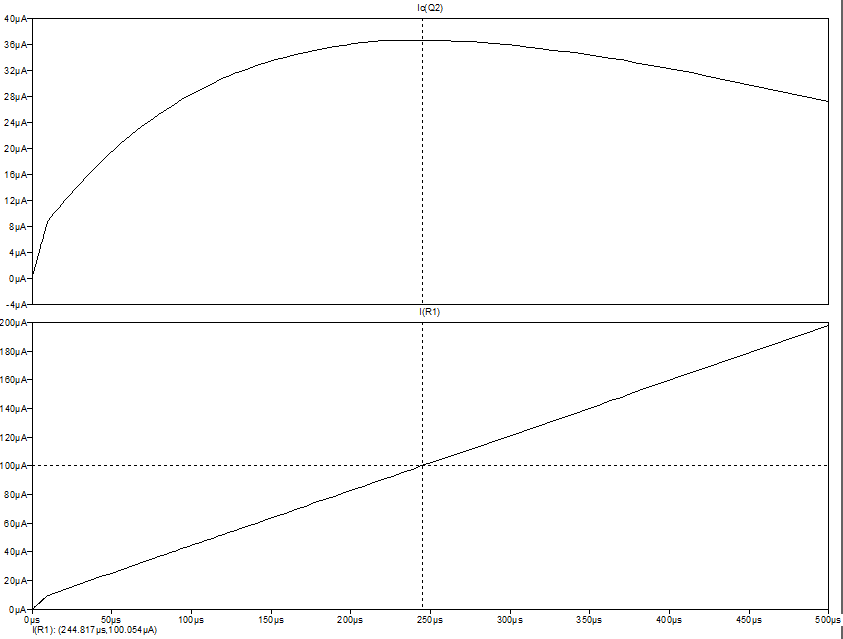# Peaking Current Source# The circuit [top]

The peaking current source is shown in the schematic below:# Application [top]

The characteristics of this type of source is that even if `\$I_{IN}\$` changes a lot `\$I_{OUT}\$` changes very little. So it is useful to generate a constant current from a changing supply where `\$I_{IN}\$` can be replaced by just a resistor.

# Design [top]

If `\$I_{IN}\$` changes then the change the change in the Vbe and the drop on the R should be equal and opposite. When that happens the current in the output transistor will remain constant since its Vbe won't change. If `\$ΔI_{IN}R={ΔI_{IN}}/g_m\$` then this condition will be fulfilled. Thus we have:
`\$\$R=V_T/I_{IN}\$\$`

Since `\$I_{IN}\$` changes there will be just 1 point in the whole `\$I_{IN}\$` range where the above relation holds and that is the point where the `\$I_{OUT}\$` curve is flat, it tapers lower on either sides.
A simulation with R=259 ohms causing the peak to be at 100uA is shown below:# Extensions [top]

The output transistor can be degenerated with a resistor like a Widlar Source to make the output current curve more flat as well.